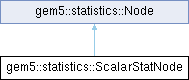gem5  v22.1.0.0
gem5::statistics::ScalarStatNode Class Reference

`#include <statistics.hh>`

Inheritance diagram for gem5::statistics::ScalarStatNode:## Public Member Functions

ScalarStatNode (const ScalarInfo *d)

const VResultresult () const
Return the result vector of this subtree. More...

Result total () const
Return the total of the result vector. More...

size_type size () const
Return the number of nodes in the subtree starting at this node. More...

std::string str () constPublic Member Functions inherited from gem5::statistics::Node
virtual ~Node ()

## Private Attributes

const ScalarInfodata

VResult vresult

## Detailed Description

Definition at line 1553 of file statistics.hh.

## ◆ ScalarStatNode()

 gem5::statistics::ScalarStatNode::ScalarStatNode ( const ScalarInfo * d )
inline

Definition at line 1560 of file statistics.hh.

## ◆ result()

 const VResult& gem5::statistics::ScalarStatNode::result ( ) const
inlinevirtual

Return the result vector of this subtree.

Returns
The result vector of this subtree.

Implements gem5::statistics::Node.

Definition at line 1563 of file statistics.hh.

References data, gem5::statistics::ScalarInfo::result(), and vresult.

## ◆ size()

 size_type gem5::statistics::ScalarStatNode::size ( ) const
inlinevirtual

Return the number of nodes in the subtree starting at this node.

Returns
the number of nodes in this subtree.

Implements gem5::statistics::Node.

Definition at line 1571 of file statistics.hh.

## ◆ str()

 std::string gem5::statistics::ScalarStatNode::str ( ) const
inlinevirtual

Implements gem5::statistics::Node.

Definition at line 1576 of file statistics.hh.

References data, and gem5::statistics::Info::name.

## ◆ total()

 Result gem5::statistics::ScalarStatNode::total ( ) const
inlinevirtual

Return the total of the result vector.

Returns
The total of the result vector.

Implements gem5::statistics::Node.

Definition at line 1569 of file statistics.hh.

References data, and gem5::statistics::ScalarInfo::result().

## ◆ data

 const ScalarInfo* gem5::statistics::ScalarStatNode::data
private

Definition at line 1556 of file statistics.hh.

Referenced by result(), str(), and total().

## ◆ vresult

 VResult gem5::statistics::ScalarStatNode::vresult
mutableprivate

Definition at line 1557 of file statistics.hh.

Referenced by result().

The documentation for this class was generated from the following file:

Generated on Wed Dec 21 2022 10:24:26 for gem5 by doxygen 1.9.1